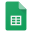# Count cell between two values in Excel and Google Sheets

To count cell numbers between two numbers you can use the COUNTIF function, for i.e. =COUNTIFS(A2:A10,">=10",A2:A10,"<=20") will count the number that is >=10 and <=20.

=COUNTIFS(A:A,">10",A:A,"<20")

### A:A = Range, Condition 1 = ">10", Condition 2 = "<20"

Check below for a detailed explanation with pictures and how to use formulas in Excel and Google Sheets.

# Count cell between two values in Excel

How to count cells between two values in Excel?# COUNT CELL BETWEEN TWO VALUES — EXCEL FORMULA AND EXAMPLE

1. =COUNTIFS(A:A,">10",A:A,"<20")

• Criteria within the formula and must be inside the quotation mark

2. =COUNTIFS(A:A,C6,A:A,D6)

• Criteria as a cell reference

• Criteria: C6 and D6

3. =COUNTIFS(A:A,C7,A:A,D7)

• Criteria as a cell reference

• Criteria: C7 and D7

# Count cell between two values in Google Sheets

How to count cells between two values in Google Sheets?# COUNT CELL BETWEEN TWO VALUES — GOOGLE SHEETS FORMULA AND EXAMPLE

1. =COUNTIFS(A:A,">10",A:A,"<20")

• Criteria within the formula and must be inside the quotation mark

2. =COUNTIFS(A:A,C6,A:A,D6)

• Criteria as a cell reference

• Criteria: C6 and D6

3. =COUNTIFS(A:A,C7,A:A,D7)

• Criteria as a cell reference

• Criteria: C7 and D7

## Wild cards and regular expressions in Excel and Google SheetsWild cards and regular expressions